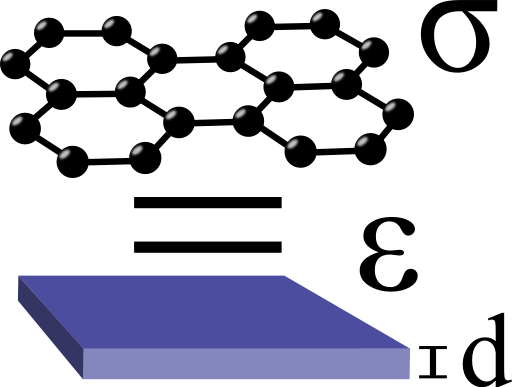## The "Permittivity" of GrapheneGraphene is a monolayer of carbon atoms and offers extremely interesting physics. Its unique properties may very well make it the silicon of the 21st century. For practical applications it is of great importance to model this material containing the main electromagnetic features. Find out in this problem how graphene can be represented as a thin layer with a certain permittivity and how this can be used for next-generation electromagnetic devices.## Problem Statement

Find the dispersion relation of transverse magnetic surface plasmon polaritons (SPPs) supported by graphene embedded in some medium with relative permittivity $$\varepsilon_{d}$$! You may want to follow these steps:

• Start from Ampère's law including the displacement current to figure out a relation between $$\varepsilon\left(\omega\right)$$ and a three-dimensional $$\sigma\left(\omega\right)=\sigma_{2D}\left(\omega\right)/d$$ assuming a certain thickness $$d$$ of graphene.
• Regard graphene now as a metallic layer with thickness $$d$$ and relative permittivity $$\varepsilon_{m}\left(\omega\right)$$
• Use the dispersion relation of transverse magnetic SPPs to find the wave-vector in the limit of vanishing thickness $$d$$.
The dispersion relation for the wave vector $$k_{p}\left(\omega\right)$$ of such an SPP supported by the given layer reads as$\begin{eqnarray*}\tanh\left\{ \sqrt{k_{p}^{2}-\varepsilon_{m}k_{0}^{2}}\frac{d}{2}\right\} & = & -\frac{\sqrt{k_{p}^{2}-\varepsilon_{m}k_{0}^{2}}\varepsilon_{d}}{\sqrt{k_{p}^{2}-\varepsilon_{d}k_{0}^{2}}\varepsilon_{m}}\ .\end{eqnarray*}$

## Background: Graphene - a Material with Tunable Conductivity

The main results for the physical quantities of graphene follow from calculations which are a little too complex to be presented here. In short, the properties of the material are related to its high conductivity $$\sigma_{2D}$$. Furthermore, $$\sigma_{2D}$$ can be tuned using the electric field effect (EFE) applying a certain voltage difference to a surrounding material. These two properties can be seen as the reason why enormous effort is put into research relating graphene at the moment.

The EFE changes the so-called chemical potential $$\mu_{c}$$ which enters the conductivity $$\sigma_{2D}$$ of graphene:$\begin{eqnarray*}\sigma_{2D}\left(\omega\right) & = & \mathrm{i}\frac{1}{\pi\hbar^{2}}\frac{e^{2}k_{B}T}{\omega+\mathrm{i}2\Gamma}\left\{ \frac{{\color{red}\mu_{c}}}{k_{B}T}+2\ln\left[\exp\left(-\frac{{\color{red}\mu_{c}}}{k_{B}T}\right)+1\right]\right\} \\& & +\mathrm{i}\frac{e^{2}}{4\pi\hbar}\ln\left[\frac{2\left|{\color{red}\mu_{c}}\right|-\hbar\left(\omega+\mathrm{i}2\Gamma\right)}{2\left|{\color{red}\mu_{c}}\right|+\hbar\left(\omega+\mathrm{i}2\Gamma\right)}\right]\ .\end{eqnarray*}$Here, $$T$$ is the temperature and $$\Gamma$$ a rate corresponding to relaxation processes. We are furthermore in the frequency (Fourier) domain with an $$\exp\left(-\mathrm{i}\omega t\right)$$ time dependence. In the literature you can find $$\mu_{c}\approx50\dots1000$$ meV and $$\Gamma\lesssim1$$ meV as characteristic values.Now we want to model this two dimensional conductivity to be able to do electrodynamic calculations, e.g. on a computer. A very decent way to do so is to find a representation of graphene as a thin three-dimensional layer with a certain permittivity supporting collective electron oscillation called surface plasmon polaritons (SPPs), see right figure. With this trick, sophisticated computations are possible and applications for graphene can be designed, see also Graphene Plasmonics: Antennas for THz Spectroscopy.

Note that the permittivity we have derived is just the dispersion relation of a kind of supported modes, transverse magnetic SPPs, not of anything that can happen! The quantity has to be used with care which I want to emphasize using the quotation marks.